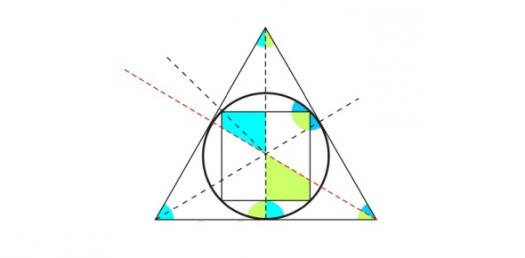# How Good Are You At Geometry?

6 Questions | Total Attempts: 31SettingsRelated Topics
• 1.
How many degrees are in a triangle?
• A.

90

• B.

360

• C.

180

• 2.
The Pythagorean theorem holds true for which type of triangle?
• A.

All triangles

• B.

Right angled triangle

• C.

Isosceles triangle

• 3.
What shape is this?
• A.

Circle

• B.

Ball

• C.

Sphere

• 4.
What is the biggest angle?
• A.

Right

• B.

Acute

• C.

Obtuse

• 5.
A polygon is a figure that is closed and has straight lines. Is this a polygon?
• A.

Yes

• B.

No

• 6.
C=2Πr (Circumference of a Circle)
• A.

True

• B.

False